## 2D transformations

CS 248 - Introduction to Computer Graphics
Autumn Quarter, 2005
Marc Levoy
Lecture notes for Thursday, October 20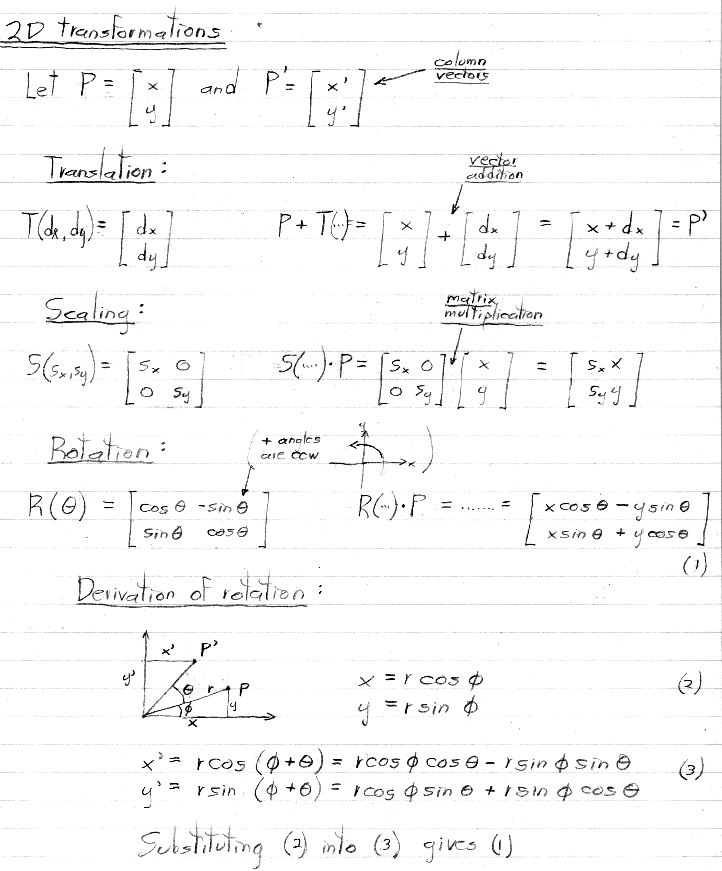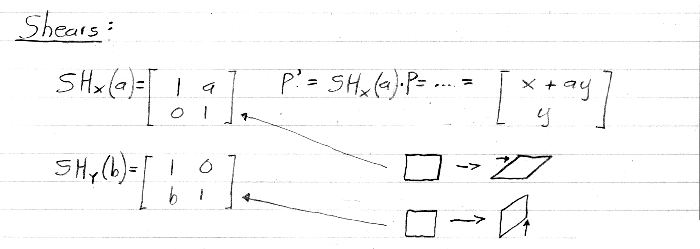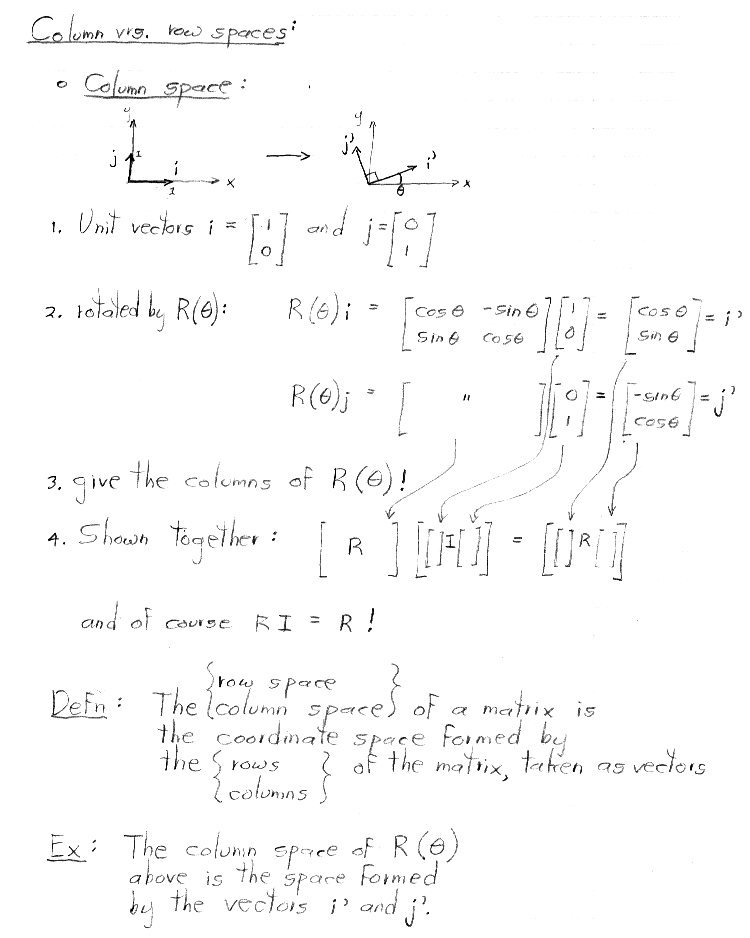These unit vectors are also called the standard basis vectors. Restating the result shown above, any matrix transforms the standard basis vectors into a set of vectors that form the columns of the matrix.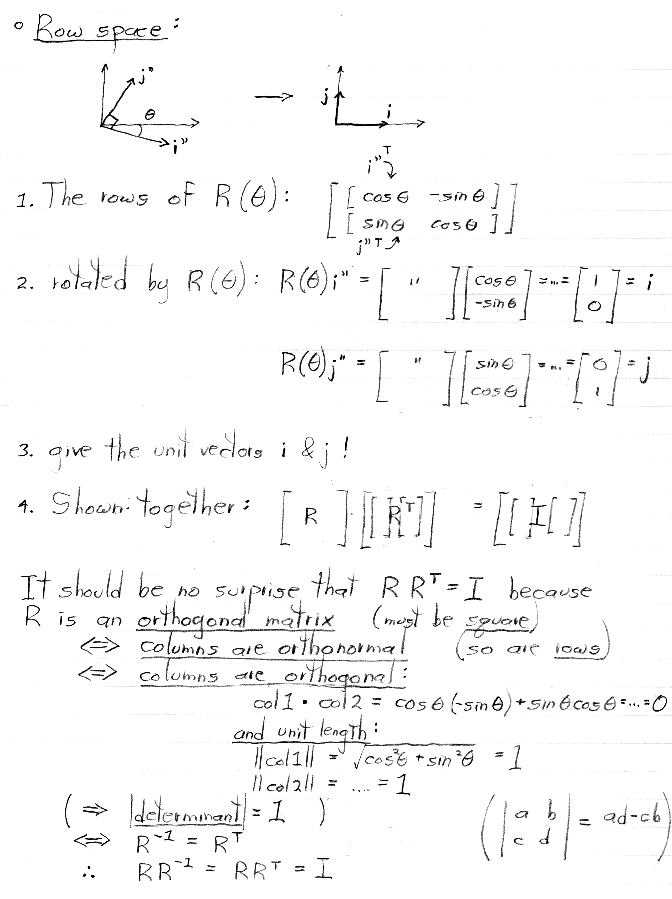In other words, an orthogonal matrix transforms its rows, taken as column vectors, into the standard basis vectors. Among the many facts listed above about orthogonal matrices, we will make particular use later in the course of the fact that the inverse of an orthogonal matrix is equal to its transpose.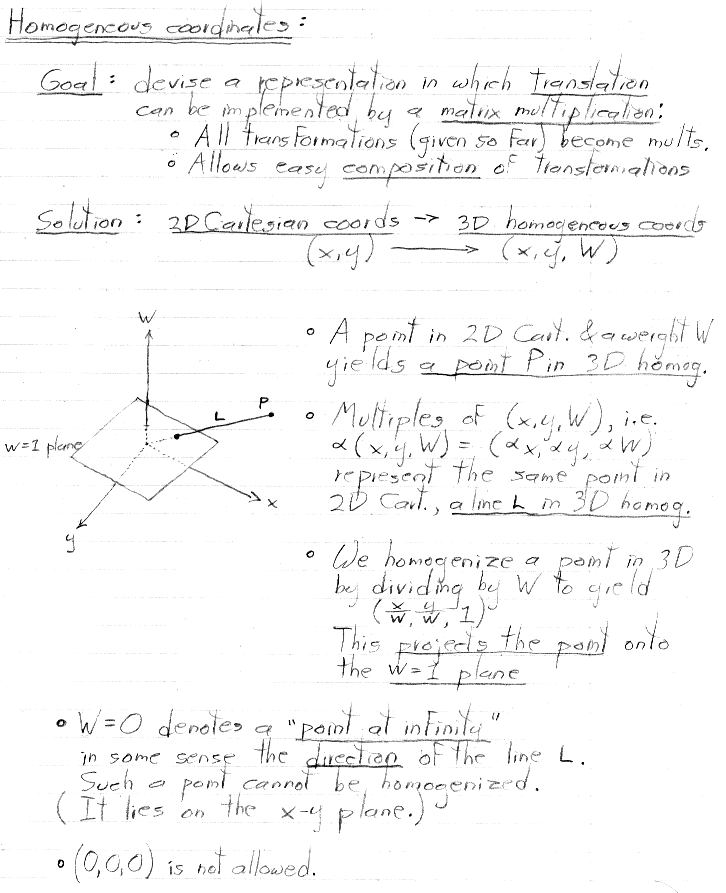Thus, the 2D Cartesian coordinates occupy the W = 1 plane in 3D homogeneous space.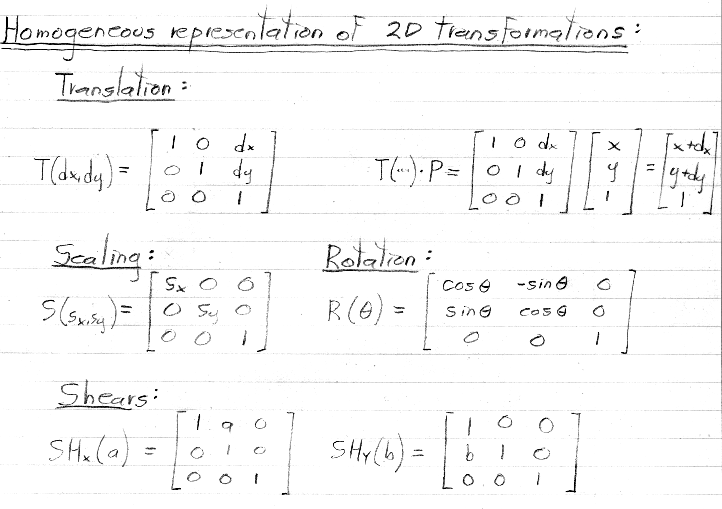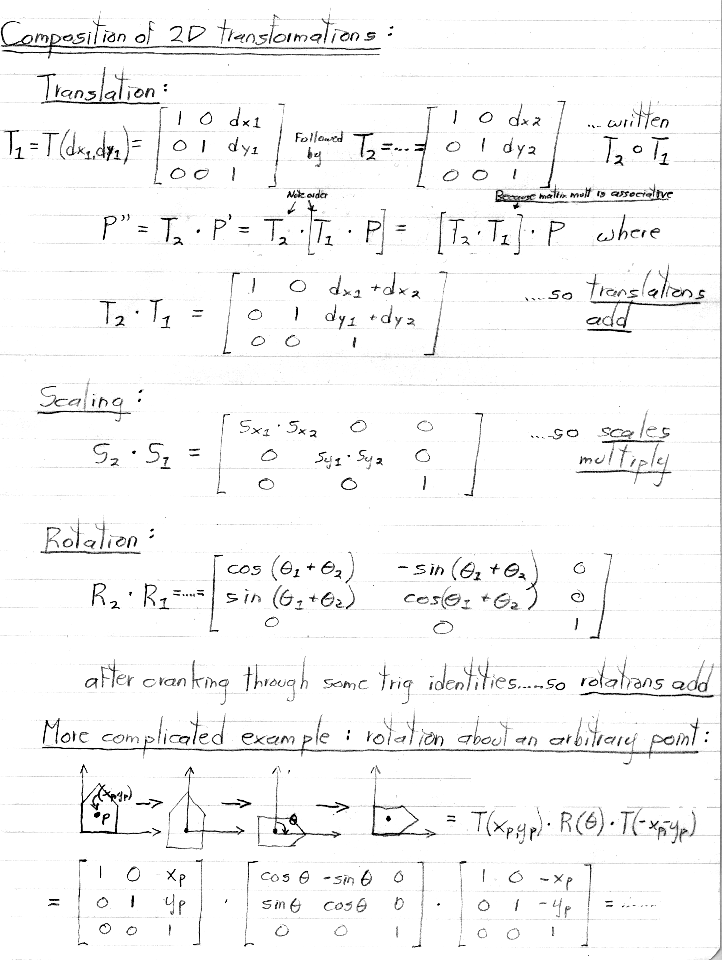levoy@cs.stanford.edu